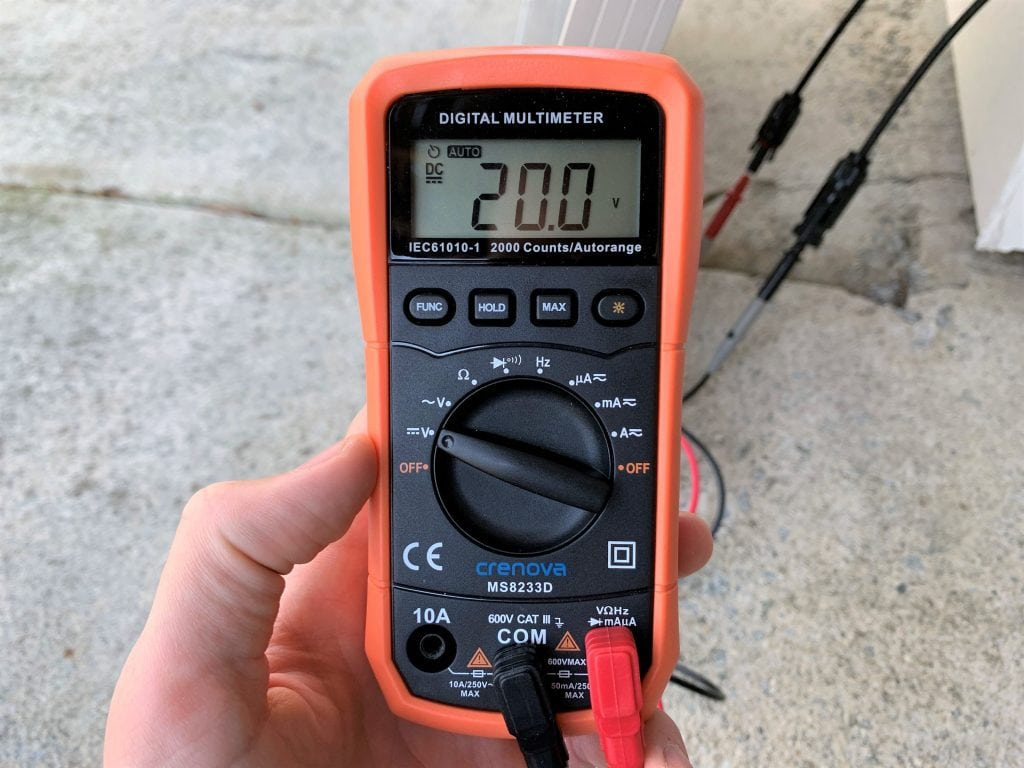# Amps to Watts (A to W) Conversion Calculator

Use our calculator to easily convert amps to watts (A to W).

Error: This field is required.
Error: This field is required.
watts
Formula: W = A × V
Formula: W = A × V × PF
Formula: W = A × V × PF × √3
Formula: W = A × V × PF × 3

Or would you rather convert watts to amps?

## Amps to Watts Conversion Tables

Here are some tables converting amps to watts at common AC and DC voltages. All the AC conversion tables assume single-phase AC with a power factor of 1.

## Converting Amps to Watts in AC vs DC Circuits

Converting amps to watts is different depending on whether you're working with direct current (DC) or alternating current (AC).

AC power is used in our modern electrical grid, as this type of electricity is easy to transmit over long distances without losses. The majority of the appliances in your home run on AC power.

DC power, on the other hand, is produced by things like batteries, fuel cells, and solar panels. The phone, tablet or laptop you are reading this on uses DC power, as these devices rely on batteries to operate.

Tip: If you're ever unsure if a device uses AC or DC, look for the following symbols: a dotted line with a solid line above it (⎓) signifies DC, while a wavy line, or tilde (~) signifies AC. You can see these symbols in the following picture of my multimeter.This multimeter is set to the DC voltage setting, as indicated by a "V" with the direct current symbol (⎓) next to it. You can see also see the AC voltage setting above it, as indicated by a "V" with the alternating current symbol (~). The current settings on the right have a wavy line with a solid line above it, indicating that those settings can measure both AC and DC current.

## How to Convert Amps to Watts for DC Circuits

To convert amps to watts in a DC circuit, simply multiply amps by volts.

Conversion formula: watts = amps × volts

Abbreviated formula: W = A × V

You'll often see this formula written slightly differently, with amps abbreviated as I instead of A and watts abbreviated as P instead of W. The formula itself remains the same, only the abbreviated version changes.

Alternate abbreviation: P = I × V

Note: This formula is sometimes called the electrical power formula, which simply states that power equals current times voltage.

### Example: DC Circuit

I have the following solar panel with a rated output of 5.41 amps at 18.5 volts.

With these specs, here's how you calculate the panel's max power output in watts:

`5.41 A × 18.5 V = 100.085 W`

So my panel is a 100 watt solar panel.

## How to Convert Amps to Watts for Single-Phase AC Circuits

To convert amps to watts in a single-phase AC circuit, multiply by watts and then by the power factor.

Conversion formula: watts = amps × volts × power factor

Abbreviated formula: W = A × V × PF

Alternate abbreviation: P = I × V × PF

### Example: Single-Phase AC Circuit

Let's say you're trying to calculate how many watts your phone charger uses. You squint at its electrical specifications and see it outputs 0.7 amps at 100-240 volts.

To figure out which voltage to use, you need to know your country's residential voltage, often called the mains voltage. Let's assume you live in the US, where the voltage of standard wall outlets is 120 volts AC.

So we have our amperage (0.7 A) and our voltage (120 V). But, because we're dealing with an AC circuit, we still need the power factor.

The power factor is a number between 0 and 1. There are some online resources that give rule-of-thumb power factors based on device type, but to find the exact power factor of a circuit, you need some equipment. I used an electricity usage monitor called a Kill-a-Watt to help me find it for this phone charger. It turned out to be 0.5.

Now we have all the numbers we need to calculate the phone charger's wattage:

`0.7 A × 120 V × 0.5 = 42 W`

It turns out your phone charger uses up to 42 watts.

If you're perceptive, you probably noticed that the output of the charger is 4.8 amps at 5 volts DC (these values are also listed on the device in the picture above). That means the max output of the charger is 24 watts (4.8 A × 5 V = 24 W). So the charger operates at around 57% efficiency (24 W ÷ 42 W ≈ 57%).

## How to Convert Amps to Watts for Three-Phase AC Circuits

The formula for calculating watts for a three-phase AC circuit depends on whether it's line to line or line to neutral voltage.

### Using Line to Line Voltage

To convert amps to watts for a three-phases AC circuit using line to line voltage, multiply amps by volts by the power factor by the square root of 3.

Conversion formula: watts = amps × volts × power factor × √3

Abbreviated formula: W = A × V × PF × √3

Alternate abbreviation: P = I × V × PF × √3

### Using Line to Neutral Voltage

To convert amps to watts for a three-phases AC circuit using line to neutral voltage, multiply amps by volts by the power factor by 3.

Conversion formula: watts = amps × volts × power factor × 3

Abbreviated formula: W = A × V × PF × 3

Alternate abbreviation: P = I × V × PF × 3

## More Electricity Calculators